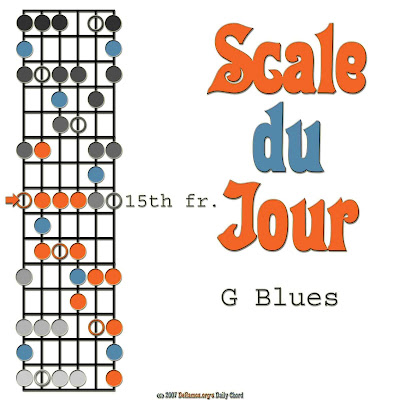## Friday, September 5, 2008

### Lesson #13: Swing Time in G Blues (Guitar, Intermediate)

To get a grasp of what it means to shuffle/swing, be sure to do the following:

1. Listen to a lot of blues and jazz records;
2. Read the Wikipedia article on the "Swung Note" for lots of background information;
3. Listen to those same blues and jazz records.

For the sake of simplicity, and due to the limitations of text lessons over audio-visual lessons, I'm going to say that a form of swing time goes: 1 and a 2 and a 3 and a 4 and a...and so on. It's a syncopated interpretation of the common time.

We'll do it over the twelve bar blues in G:

G and a 2 and a 3 and a 4 and a
G and a 2 and a 3 and a 4 and a
G and a 2 and a 3 and a 4 and a
G and a 2 and a 3 and a 4 and a
C and a 2 and a 3 and a 4 and a
C and a 2 and a 3 and a 4 and a
G and a 2 and a 3 and a 4 and a
G and a 2 and a 3 and a 4 and a
D and a 2 and a 3 and a 4 and a
C and a 2 and a 3 and a 4 and a
G and a 2 and a 3 and a 4 and a
G and a 2 and a C C# D yeah...

When you have that down (and its myriad variations), try to play some bluesy lead guitar in G blues: